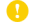ばね論文集
Online ISSN : 1348-1479
Print ISSN : 0385-6917
ISSN-L : 0385-6917

ジャーナル フリー

2017 年 2017 巻 62 号 p. 37-47J-STAGE : trbane 2018 63 pp.103-104

In this study, 3D finite element analyses have been made on the stress intensity factors for semi-elliptical surface cracks on the wire surface of a tension coil spring in order to investigate the effects of spring parameters on the correction factors, Fi (i=I, II and III) for the three modes of the stress intensity factors. The parameters selected for the present analyses are crack inclination angle θ, crack position on wire surface, normalized half crack surface length c/r, crack aspect ratio a/c and spring index s=R/r where c is the half crack surface length, r the wire radius, a the crack depth and R the coil radius of the spring. The mode I correction factor, or the normalized mode I stress intensity factor FI was found dominant over the correction factors for the other two modes in all the cases investigated. The most influential parameter for FI was the crack aspect ratio a/c, and the largest FI value was calculated as approximately 2.75 for a/c=4 near wire surface, i.e., φ=0°. The most influential parameter for the other correction factors FII and FIII was the crack inclination angle θ. The correction factors FII and FIII were revealed to take on smaller maximum values than FI: i.e., the maximum absolute value of FII was found approximately 1.3 for θ=0° and 90°near wire surface (φ=0° and 180°), and that of FIII approximately 0.59 for θ=0° and 90°at the deepest point of the crack (φ=90°).著者関連情報